## Sunday, July 6, 2014

### Volume of a Bottle (Approximate)

This blog entry will deal with finding two formulas (approximate?) for finding the volume of a bottle. This includes plastic water bottles, beer bottles, and carry bottles.

In order to know how to find the volume, let's loom at the basic parts. This was accomplished by a basic search on Google: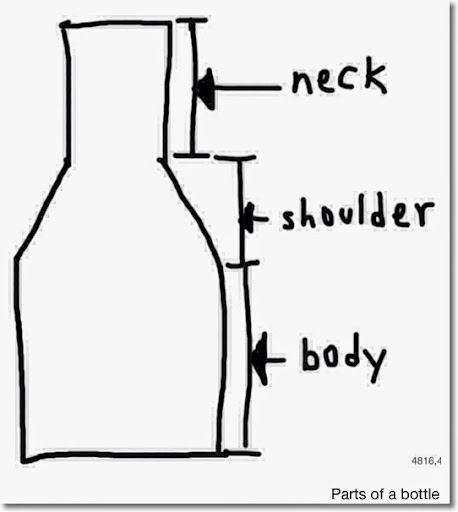Assume that the neck and body are cylinders. The shape of the shoulder is going to vary. First, let's work with a bottle with a linear shoulder, and one with a parabolic shoulder. The volume of the bottle will be measured in three parts.

V = VN + VS + VB

Where:
VN = volume of the neck
VS = volume of the shoulder
VB = volume of the body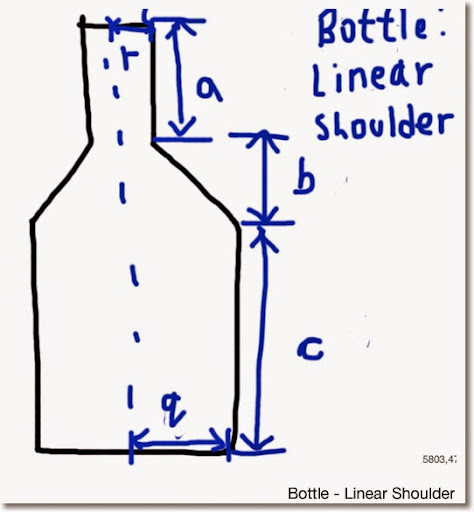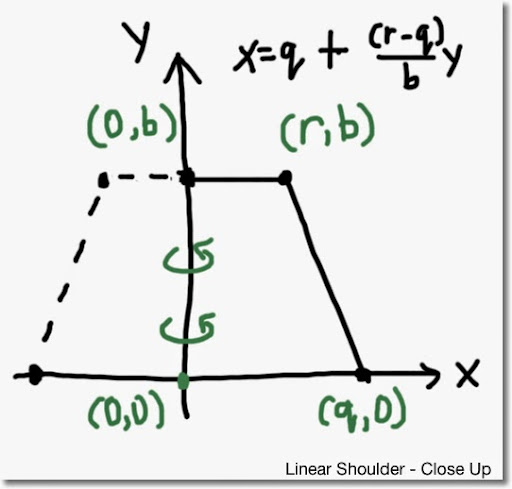Bottle - Linear Shoulder:

Neck: VN = π * r^2 * a

Body: VB = π * q^2 * c

Shoulder:

Let's use the technique of the Method of Discs.

Top Boundary: y = b

Bottom Boundary: y = 0

Left Boundary: x = 0

Right Boundary: x = (r - q)/b * y + q

The line between the points (r, b) and (q, 0).

Slope:
Δy/Δx = (b - 0)/(r - q) = b/(r - q)

Y Intercept
y = b/(r - q) * x + β

Use point (q, 0) (x = q and y = 0)
0 = b/(r - q) * q + β
β = -b/(r - q) * q

Solving for x:
y = b/(r - q) * x - b/(r - q) * q
(r - q)/b * y = b * x - b * q
x = (r - q)/b * y + q

Volume of the Shoulder:
VS =

b
∫ ((r - q)/b * y + q)^2 dy * π =
0

b
∫ (r - q)/b * ((r - q)/b * y + q)^2 dy * (π * b)/(r - q) =
0

b
[ 1/3 * ((r - q)/b * y + q)^3 ] * (π * b)/(r - q) =
0

(π * b)/(3 * (r - q)) * (r^3 - q^3) =

(π * b)/(3 * (r - q)) * (r - q) * (r^2 + r * q + q^2) =

(π * b)/3 * (r^2 + r * q + q^2)

Total Volume - Bottle: Linear Shoulder:

V = VN + VS + VB =

π * r^2 * a + (π * b)/3 * (r^2 + r * q + q^2) + π * q^2 * c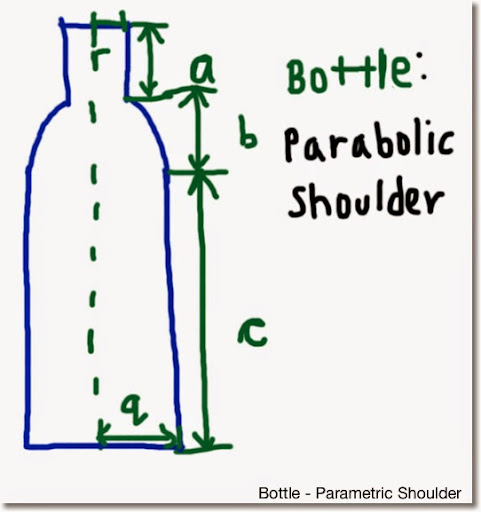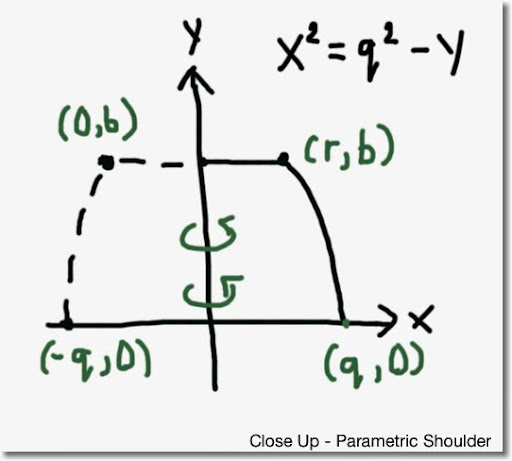Bottle: Parabolic Shoulder

Neck: VN = π * r^2 * a

Body: VB = π * q^2 * c

Shoulder:

Let's use the technique of the Method of Discs.

Top Boundary: y = b

Bottom Boundary: y = 0

Left Boundary: x = 0

Right Boundary:

Parabolic Equation with roots x = -q and x = q and the curve concave downward, an equation to describe this curve can be:

y = -x^2 + q^2
y + x^2 = q^2
x^2 = q^2 - y (note we have x^2)

Volume of the Shoulder:
VS =

b
∫ q^2 - y dy * π =
0

b
[ q^2 * y - y^3/3 ] * π =
0

π * (b * q^2 - b^3/3)

Total Volume - Parabolic Shoulder:

V = VB + VS + VN =

π * q^2 * c + π * (b * q^2 - b^3/3) + π * r^2 * a

Example:
a = 1 in, b = 1 in, c = 4 in, r = 0.9 in, q = 1.5 in
V = π * 1.5^2 * 4 + π * (1 * 1.5^2 - 1^3/3) + π * 0.9^2 * 1 ≈ 36.84041 in^3

These are two ways to approximate the volume of the bottles.

Eddie

This blog is property of Edward Shore. 2014

#### 3 comments:

1.Very interesting blog post, good job and thanks for sharing such a good blog.read more @ ball volume calculator
moderncalculator.com
know more
Moderncalculator

2.See the how calculate volumes in WebCalc - http://webcalc.com.br

3.Can't get the understanding

### Swiss Micros DM16L: Advanced Boolean and Factorial (up to 20)

Swiss Micros DM16L:   Advanced Boolean and Factorial (up to 20) Introduction The program listing, for the Swiss Micros DM16L and Hewlett Pac...